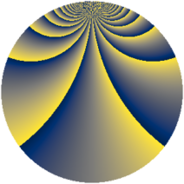# Properties

 Label 1610.2.btLevel $1610$ Weight $2$ Character orbit 1610.bt Rep. character $\chi_{1610}(37,\cdot)$ Character field $\Q(\zeta_{132})$ Dimension $3840$ Sturm bound $576$

# Related objects

## Defining parameters

 Level: $$N$$ $$=$$ $$1610 = 2 \cdot 5 \cdot 7 \cdot 23$$ Weight: $$k$$ $$=$$ $$2$$ Character orbit: $$[\chi]$$ $$=$$ 1610.bt (of order $$132$$ and degree $$40$$) Character conductor: $$\operatorname{cond}(\chi)$$ $$=$$ $$805$$ Character field: $$\Q(\zeta_{132})$$ Sturm bound: $$576$$

## Dimensions

The following table gives the dimensions of various subspaces of $$M_{2}(1610, [\chi])$$.

Total New Old
Modular forms 11840 3840 8000
Cusp forms 11200 3840 7360
Eisenstein series 640 0 640

## Trace form

 $$3840q + 16q^{6} + O(q^{10})$$ $$3840q + 16q^{6} - 192q^{16} + 72q^{18} + 68q^{23} + 8q^{25} - 8q^{26} - 88q^{28} + 8q^{31} + 8q^{35} - 368q^{36} + 88q^{37} + 32q^{41} - 16q^{46} + 8q^{47} + 44q^{56} - 176q^{57} + 352q^{61} + 48q^{62} - 80q^{70} - 16q^{71} - 16q^{72} - 56q^{73} + 96q^{77} - 64q^{78} - 160q^{81} + 32q^{82} + 136q^{85} - 44q^{86} + 112q^{87} + 40q^{92} - 8q^{93} + 140q^{95} + 8q^{96} - 168q^{98} + O(q^{100})$$

## Decomposition of $$S_{2}^{\mathrm{new}}(1610, [\chi])$$ into newform subspaces

The newforms in this space have not yet been added to the LMFDB.

## Decomposition of $$S_{2}^{\mathrm{old}}(1610, [\chi])$$ into lower level spaces

$$S_{2}^{\mathrm{old}}(1610, [\chi]) \cong$$ $$S_{2}^{\mathrm{new}}(805, [\chi])$$$$^{\oplus 2}$$